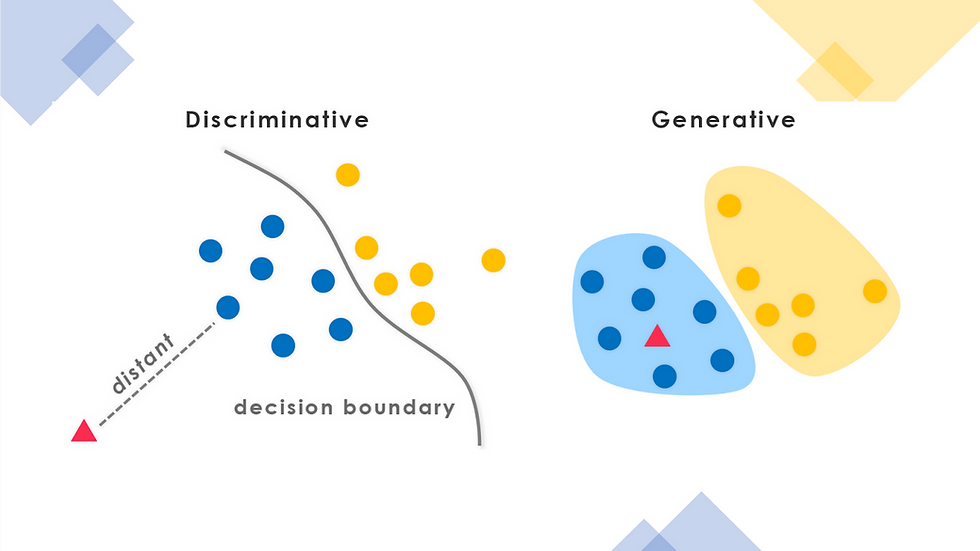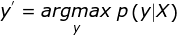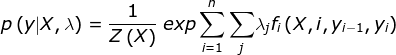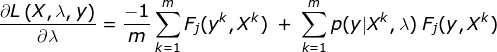top of page
Search

# Conditional Random FieldsWhat is the difference between ‘India’ and ‘India Times’? Obviously, we all know that ‘India’ is the name of a country and ‘India Times’ is the name of a newspaper. Another question: how did you know that? At some point of time in your life you were introduced to these words i.e. you learnt them and now when the question was asked you remembered what you learnt i.e. you knew the context.

How does these questions relate to CRFs (Conditional Random Fields)?

First we will discuss about generative and discriminative models and then we will talk about CRFs.

## GENERATIVE Vs. DISCRIMINATIVE

Generative Algorithms model the actual distribution of the classes. Discriminative Algorithms model the decision boundary between classes. To understand it better, let us consider a very common example: Suppose you were asked to classify a speech to a language. There are two possible ways to do it:

1. You learn each language there is and then classify the speech using what you learnt

2. You just learn the differences between the languages without learning them from scratch and then classify the speech accordingly

The first approach is called a generative approach, it learns how the data is generated and then classifies and the second approach is called a discriminative approach as it just learns the decision boundaries and then classifies.A Generative approach model is time consuming and thus discriminative approach models are preferred when we have a sufficiently large amount of data but when the data is scarce the generative approach is better suited.

We now know what generative and discriminative models are.

## Conditional Random Field

Since we are introducing a complex topic we will consider an example to get a better understanding. Imagine that you have a sequence of words and you have to tag the words with the part of speech that they represent. You can do this in two ways:

First, by ignoring the sequential aspect and tagging the words according to the tags in the train set of the corpus. In this method the tag assigned to a word will be the same as the tag that corresponds to the word in the train set. But, we know that a word can represent different parts of speech, for e.g. the word ‘Will’ can be a modal verb or a noun depending on the context. Ignoring the sequential aspect of the words makes us lose the context. Hence, we are not able to effectively assign tags to words.

The above problem can be overcome if we take the sequential aspect into account as well along with the classification. In this method the tag assigned to a word depends on the tag that corresponds to the word in the train set and on the previous tag in the sequence. This way we gain context and we can correctly classify the word ‘Will’ as a modal verb or a noun depending on the place of the word in the sequence.

The second approach is achieved by the use of Conditional Random Fields.

Let us define CRFs:

Conditional Random Fields are a discriminative model which is used for predicting sequences. They use contextual information from previous labels, thereby increasing the amount of information the model has to make a good prediction.

Mathematical overview:

Since Conditional Random Fields are a discriminative model, we calculate the conditional probability i.e.

## p (y | X)

It is the probability of the output vector y given an input sequence X. To predict the proper sequence we need to maximize the probability and we take the sequence with maximum probability.We will use a feature function f.The output sequence is modelled as the normalized product of the feature function.Where Z (X) is the normalization.λ (lambda) is the feature function weights, which is learned by the algorithm. For the estimation of the parameter λ, we will use maximum likelihood estimation. Thus the model is log-linear on the feature function.Next, we apply partial derivative w.r.t. λ in order to find argmin on the negative log function.For the parameter optimization, we use iterative approach i.e. gradient descent based method. The gradient update step for the CRF model is:Thus, we use Conditional Random Fields by first defining the feature functions needed, then initializing the weights to random values, and then applying Gradient Descent iteratively until the parameter values (lambda) converge.

Applications of CRFs:

CRF are widely used in NLP for various purposes two of which are POS tagging and Named Entity Recognition.

Other processes which utilise CRFs are gene prediction, activity and gesture etc.

Note:

If you need implementation for any of the topics mentioned above or assignment help on any of its variants, feel free contact us on contact@codersarts.com.